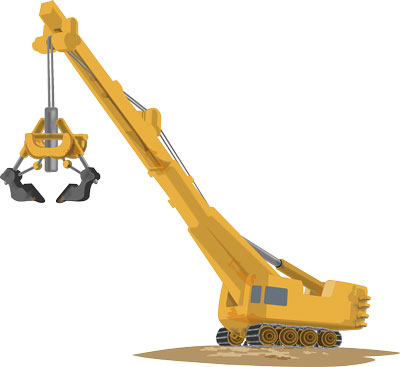## What is Power?

In physics, power is the rate of doing work. Because work does not deal with time, it only tells us that how much energy is consumed in performing the work.

The work can be done at different rates. For example, you and your friend are pushing the boxes having the same weight. Now, your friend reaches the target in 60 seconds and you reach the same target in 100 seconds. Both of you have expanded the same amount of energy to push the box. But, your friend did the work faster than you, so its power was more than you.

## How is Power Measured?

Power is measured in watts in the international system of units. One watt of power is equal to one joule energy expanded in one second. The mathematical formula for calculating the power is:

Power = Work/time
P = W/t

Example: Two persons are carrying boxes towards their home. The first person is reaching the home in 100 seconds, whereas the second person in 40 seconds. Calculate the power of both persons, if the work performed by both is 400 joules.

Solution:

Power of the first person is:

P1 = work / time = 400 joules / 100 seconds = 4 watts

Power of the second person:

P2 = work / time = 400 joules / 40 seconds = 10 watts

Clearly, the second person is doing the work with more power than the first person.

The power is also measured in another unit, which is called horsepower. Horsepower is bigger unit than a watt and is mostly used for heavy duty machines. One horsepower is equal to 745.7 watts.

## Electrical PowerOur rate of electricity consumption is also measured in watts. To find out how much power we are using, just multiply the current flowing in the circuit with the voltage of the circuit.

P = Voltage*Current

## Facts

• Chemicals that release energy very fast result into the explosions.
• Your electricity bill is calculated in kilowatt-hours. This is the unit of energy, not the power.
• In real practice, machines with high power consume more energy than the machines with low power to perform the same Because increasing power can decrease the efficiency.